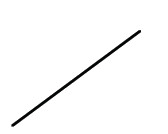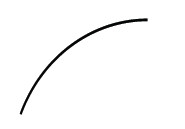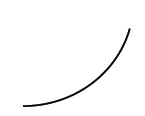### Home > CALC > Chapter 1 > Lesson 1.2.4 > Problem1-76

1-76.

Each of the continuous functions in the table below is increasing, but each increases differently. Match each graph below with the function that grows in a similar fashion in the table.

$x$

$1$

$2$

$3$

$4$

$5$

$6$

$7$

$8$

$9$

$f(x)$

$15.5$

$19.0$

$22.5$

$26.0$

$29.5$

$33.0$

$36.5$

$40.0$

$43.5$

$g(x)$

$1$

$2$

$4$

$8$

$16$

$32$

$64$

$128$

$256$

$h(x)$

$12$

$76$

$108$

$124$

$132$

$136$

$138$

$139$

$139.5$

1.1.1.Use the eTool below to create the graphs of the functions.
Click the link at right for the full version of the eTool: Calc 1-76 HW eTool